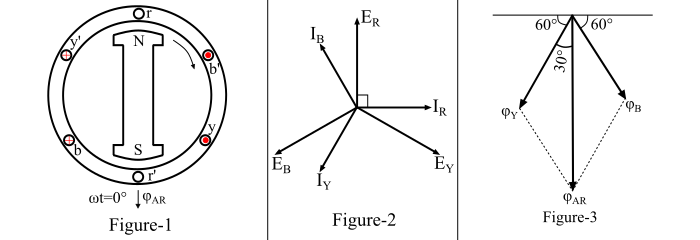# Armature Reaction in Alternator at Lagging Power Factor Load

Consider a 2-pole alternator as shown in Figure-1. Suppose that the alternator is loaded with an inductive load of zero power factor lagging. In this case, the phase current 𝐼𝑅 , 𝐼𝑌 and 𝐼𝐵 will be lagging with their respective phase voltages 𝐸𝑅 , 𝐸𝑌 and 𝐸𝐵 by 90°. The phasor diagram of the alternator is shown in Figure2.Now, at time t = 0, the instantaneous values of currents and fluxes are given by,

$$\mathrm{𝑖_{𝑅} = 0;\:\:φ_{𝑅} = 0}$$

$$\mathrm{𝑖_{𝑌} = 𝐼_{𝑚}\:sin(−120°) = −\frac{\sqrt{3}}{2}𝐼_{𝑚};\:\:\:φ_{𝑌} = −\frac{\sqrt{3}}{2}φ_{𝑚}}$$

$$\mathrm{𝑖_{𝐵} = 𝐼_{𝑚}\:sin(120°) = \frac{\sqrt{3}}{2}𝐼_{𝑚};\:\:\:φ_{𝐵} = \frac{\sqrt{3}}{2}φ_{𝑚}}$$

The space diagram of the magnetic fluxes is shown in Figure-3. By the parallelogram law, the resultant armature reaction flux is given by,

$$\mathrm{𝜑_{𝐴𝑅} =\sqrt{φ^{2}_{𝑌} + φ^{2}_{B} + 2 φ_{𝑌}\:φ_{𝐵}\:cos\:60°}}$$

$$\mathrm{\Rightarrow\:φ_{𝐴𝑅} =\sqrt{(\frac{\sqrt{3}}{2}φ_{𝑚})^{2}+(\frac{\sqrt{3}}{2}φ_{𝑚})^{2}+2 \times (\frac{\sqrt{3}}{2}φ_{𝑚})\times (\frac{\sqrt{3}}{2}φ_{𝑚}) \times (\frac{1}{2})}}$$

$$\mathrm{∴\:𝜑_{𝐴𝑅} = 1.5\:φ_{𝑚}}$$

Here, it can be seen that the direction of the armature reaction flux φ𝐴𝑅 is opposite to the main field flux. Therefore, it will oppose and weaken the main field flux. This effect of the armature reaction is said to be demagnetising. Again it can also be shown that for successive positions of the rotor, the armature reaction flux φ𝐴𝑅 remains constant in magnitude equal to 1.5 𝜑𝑚 and rotates at synchronous speed. Also, the EMF induced in each phase by the armature reaction flux lag the respective phase currents by 90°.

Therefore, when the alternator supplies a load at lagging power factor, then the effect of the armature reaction is partly demagnetising and partly cross-magnetising.